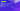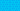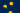Pricing# What is interest rate parity (IRP)?

Written by

Both interest rates and currency rates vary from one country to the next. Interest rate parity is a theory that helps resolve the balance between these two figures when investing. Here’s a closer look at the interest rate parity formula and why it matters.

## What is interest rate parity?

Interest rate parity (IRP) is an equation used to manage the relationship between currency exchange and interest rates. It’s used by investors, playing a pivotal role in connecting spot exchange rates, foreign exchange rates, and interest rates on the foreign exchange markets.

The fundamental concept behind the IRP is that the interest rate differential between two countries will be equal to the differential between the spot exchange rate and the forward exchange rate. When you invest in different currencies, the hedged returns should be the same regardless of fluctuations in interest rates.

The forward exchange rate should equal the spot currency exchange rate multiplied by the interest rate of the home country, then divided by the foreign currency interest rate.

Interest rate parity is also behind the no-arbitrage concept. In foreign exchange markets, this refers to the purchase and sale of a single asset enabling a trader to benefit from price differences. In other words, a forex trader can’t lock in the currency exchange rate from one country at a lower price, simultaneously purchasing another currency with a higher interest rate.

## Covered vs. uncovered interest rate parity

When the no-arbitrage condition mentioned above is satisfied using forward contracts, the IRP is ‘covered.’ If the no-arbitrage condition can still be met without using forward contracts to hedge against risk, this is called uncovered interest rate parity.

Uncovered and covered interest rate parity look similar, the only difference being the use of forward contracts. For example, imagine that a British investor is converting AUD into GBP. The investor agrees to invest the foreign currency (AUD in this case) locally at a risk-free foreign rate. He enters a forward rate agreement, which states he will convert any proceeds at the end of the investing period into GBP at the forward exchange rate. This would be a covered interest rate parity example concept.

By contrast, he could also choose to convert the AUD into GBP using the spot exchange rate, then investing this GBP for the same period at the local risk-free rate. This technique would be using uncovered interest rate parity, and both should end up with equal cash flows.

## Interest rate parity example

To give another covered interest rate parity example, imagine that U.S. Treasury bills offer an annual interest rate of 1.25%, while Australian Treasury bills are offering a 0.75% annual interest rate. If an Australian investor wanted to take advantage of the higher U.S. interest rates, they would have to exchange AUD for USD to purchase the Treasury bill.

After this, if the same investor wished to sell a one-year forward contract on the USD, using covered IRP, the transaction could only have a return of 0.75%. This would ensure the transaction meets the no-arbitrage condition of interest rate parity.

## Interest rate parity formula

Before looking at the interest rate parity formula, it’s helpful to explain the terminology used.

Spot exchange rates are the current exchange rates, while forward exchange rates refer to the future exchange rate of a currency. Banks and currency dealers offer forward rates from days to years, quoted at a bid-ask spread.

The difference between a spot rate and forward rate is called a swap point. When the difference is positive, it’s called a forward premium. A negative difference is a forward discount. When a currency with lower interest rates is compared to one with higher rates, it trades at a forward premium.

There are different interest rate parity equations for covered and uncovered IRP. Covered IRP shows the forward exchange rate; uncovered IRP shows the spot exchange rate.

Here’s the uncovered interest rate parity formula:

ST(a/b) = St(a/b) * (1+ ia) / (1 + ib)

The covered interest rate parity formula looks like this:

Ft(a/b) = St(a/b) * (1+ ia)T / (1 + ib)T

In both cases, here are what the components of the equation stand for:

• ST(a/b) = The Spot Rate

• St(a/b) = Expected Spot Rate at time T

• Ft(a/b) = The Forward Rate

• T = Time to Expiration Date

• ia = Interest Rate of Country A

• ib = Interest Rate of Country B

If you run the equation and the IRP doesn’t hold true, then it might be possible to use another arbitrage strategy.

## We can help

GoCardless helps you automate payment collection, cutting down on the amount of admin your team needs to deal with when chasing invoices. Find out how GoCardless can help you with ad hoc payments or recurring payments.

Interested in automating the way you get paid? GoCardless can help

Contact salesSales

Contact sales

+1(415) 523-2279

Support

help@gocardless.com